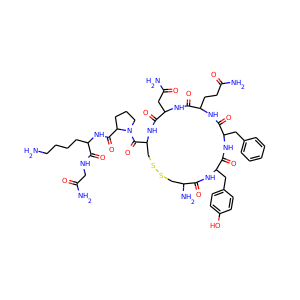877-202-0205     support@chemchart.com     @chemchartLysine vasopressin
Weight 1056.239 g/mol C46H65N13O12S2 16 13 2 19

# Lysine vasopressin (50-57-7)

## Lypressin  ·  Diapid  ·  8-Lysine VasopressinScore: #948 in Neuroscience , #1962 in Biochemistry , #4150 in Biology , #5234 in Chemistry
"What do you need help with?"### Alternate Names

• Lypressin
• Diapid
• 8-Lysine Vasopressin
• Lys Vasopressin
• 8 Lysine Vasopressin
• Lysyl Vasopressin
• Postacton
• Lys-Vasopressin
• SMILES
C1CC(N(C1)C(=O)C2CSSCC(C(=O)NC(C(=O)NC(C(=O)NC(C(=O)NC(C(=O)N2)CC(=O)N)CCC(=O)N)CC3=CC=CC=C3)CC4=CC=C(C=C4)O)N)C(=O)NC(CCCCN)C(=O)NCC(=O)N
• InChIKey